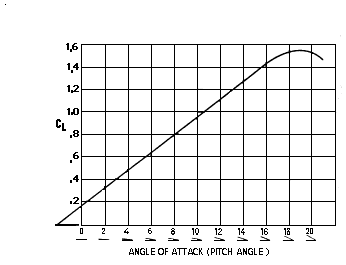## Pengalaman Belajar Fisika di SMAN BI 1 Banjar Ada Apa Dengan Fisika? Lift Formula

SUBJECT: Aeronautics
TOPIC: Lift
DESCRIPTION: A set of problems dealing with the aerodynamic lift equation.
CONTRIBUTED BY: Carol Hodanbosi
EDITED BY: Jonathan G. Fairman - August 1996

#### Purpose:

To understand for lift formula that determines aircraft lift capabilities.

#### Concept:

An aircraft's lift capabilities can be measured from the following formula:
L = (1/2) d v2 s CL
• L = Lift, which must equal the airplane's weight in pounds
• d = density of the air. This will change due to altitude. These values can be found in a I.C.A.O. Standard Atmosphere Table.
• v = velocity of an aircraft expressed in feet per second
• s = the wing area of an aircraft in square feet
• CL = Coefficient of lift , which is determined by the type of airfoil and angle of attack.
The angle of attack and CL are related and can be found using a Velocity Relationship Curve Graph (see Chart B below).

#### Exercises

1. You are flying an F-117A fully equipped, which means that your aircraft weighs 52,500 pounds. You want to maintain equilibrium in straight and level flight at an altitude of 30,000 feet, cruising at 400 knots to conserve fuel. The aircraft's wing area is 1,140 square feet. At what angle of attack should the F-117A be set to maintain equilibrium? ** Additional information you need to know to solve this problem:
How are knots related to mph to ft/s? See conversion below
What is air density at 30,000 feet? See following chart (I.C.A.O.). chart A
What is the relationship between CL and angle of attack?
Use following Chart B to determine this value.1 knot = 1 nautical mile per hour = 6,076 ft per hour
1 mph = 1 mile per hour = 5,280 feet per hour
2. To maintain an altitude of 45,000 feet at a constant speed requires an angle of attack of 4° . Your F-22 weighs 56,450 pounds, with a wing area of 830 square feet. To stay in level flight , what should your air speed indicate? The new F-22 air speed is indicated in Mach speed, not in knots. ** Additional information you need to know to solve this problem:
How does Mach speed convert from feet per second or from knots?
An angle of attack of 4° converts to what CL?
What is the density of air at 45,000 feet?
Mach 1 is the speed of sound, which varies with the air density. See the following I.C.A.O. chart (chart A) below. Mach 2 is twice the speed of sound, Mach 3 is three times the speed of sound.
Use the Velocity Relationship Curve Graph chart below, Chart B, to find the appropriate CL from the given angle of attack.
3. At what velocity should you be traveling in the F-22 to maintain an 0° angle of attack at an altitude of 42,000 feet? Your weight is 57,600 pounds
4. Using the same plane (F-22) the same weight (57,600 pounds) and angle of attack, what should be its cruising speed at 36,000 feet? While descending from 42,000 feet to 36,000 feet the plane covers a horizontal distance of 40 nautical miles. What is the decent angle in degrees?

Chart A
I.C.A.O. Standard Atmosphere Table
```
Altitude          Density     Speed of Sound
(Feet)             (d)         (Knots)
0          .002377          661.7
1,000          .002308          659.5
2,000          .002241          657.2
3,000          .002175          654.9
4,000          .002111          652.6
5,000          .002048          650.3
6,000          .001987          647.9
7,000          .001927          645.6
8,000          .001868          643.3
9,000          .001811          640.9
10,000          .001755          638.6
15,000          .001496          626.7
20,000          .001266          614.6
25,000          .001065          602.2
30,000          .000889          589.5
35,000          .000737          576.6
36,089*         .000706          573.8
40,000          .000585          573.8
45,000          .000460          573.8
50,000          .000362          573.8
55,000          .000285          573.8```
* Geopotential of Tropopause

Chart B
Velocity Relationship Curve Graph